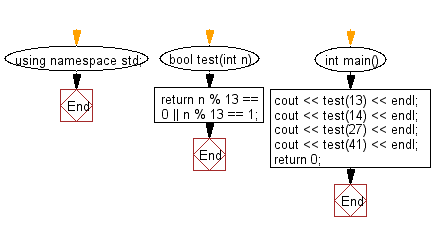﻿ C++ : Check if a non -ve number is a multiple of 13 or more# C++ Exercises: Test if a given non-negative number is a multiple of 13 or it is one more than a multiple of 13

## C++ Basic Algorithm: Exercise-39 with Solution

Write a C++ program to test if a given non-negative number is a multiple of 13 or it is one more than a multiple of 13.

Sample Solution:

C++ Code :

``````#include <iostream>

using namespace std;

bool test(int n)
{
return n % 13 == 0 || n % 13 == 1;
}

int main()
{
cout << test(13) << endl;
cout << test(14) << endl;
cout << test(27) << endl;
cout << test(41) << endl;
return 0;
}
``````

Sample Output:

```1
1
1
0
```

Flowchart:C++ Code Editor: